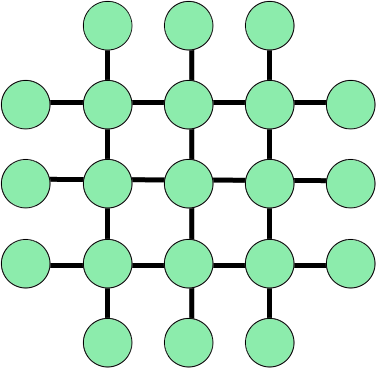BrainDen.com - Brain Teasers
• 0

# Equal sum of numbers

## QuestionPut number 1 to 21 to the circles, so sum of the numbers in circles at each line are equal.

## Recommended Posts

• 0

Simple:

Spoiler

The center 3x3 is any normal magic square of order three. (The numbers 1 to 9, with sum 15 on each row/column)

The outer circles are the numbers 10 to 21 in pairs with sum 31. (10+21 ... 15+16)

The sum of the numbers in each line is then 46.

Alternately:

Spoiler

The center 3x3 is a magic square using the numbers 7 to 15 with row/column sum of 33, with the remaining numbers in the outer circles having pairwise sum of 22. The sum of the numbers in each line is then 55.

Or the center 3x3 is a magic square using the numbers 13 to 21 with row/column sum of 51, with the remaining numbers in the outer circles having pairwise sum of 13. The sum of the numbers in each line is then 64.

Question:

Spoiler

Is there a solution such that the center 3x3 is not a magic square?

## Join the conversation

You can post now and register later. If you have an account, sign in now to post with your account.×   Pasted as rich text.   Paste as plain text instead

Only 75 emoji are allowed.

×   Your previous content has been restored.   Clear editor

×   You cannot paste images directly. Upload or insert images from URL.